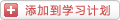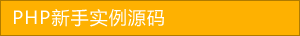﻿ 详解PHP的反射使用 - php高级应用 - PHP粉丝网

# 详解PHP的反射使用

##### 发布:smiling　来源: PHP粉丝网 　添加日期：2022-06-30 10:57:01　浏览: 评论：0

1. /**
2.
3.  * 学生类
4.
5.  *
6.
7.  * 描述信息
8.
9.  */
10.
11. class Student
12.
13.
14.     const NORMAL = 1;
15.
16.     const FORBIDDEN = 2;
17.
18.     /**
19.
20.      * 用户ID
21.
22.      * @var 类型
23.
24.      */
25.
26.     public \$id
27.
28.     /**
29.
30.      * 获取id
31.
32.      * @return int
33.
34.      */
35.
36.     public function getId()
37.
38.     {
39.
40.         return \$this->id;
41.
42.     }
43.
44.     public function setId(\$id = 1)
45.
46.     {
47.
48.         \$this->id = \$id
49.
50.     }
51.
52.
53. \$ref = new ReflectionClass('Student');
54.
55. \$doc = \$ref->getDocComment();
56.
57. echo \$ref->getName() . ':' . getComment(\$ref) , "\n"
58.
59. echo "属性列表：\n"
60.
61. printf("%-15s%-10s%-40s\n"'Name''Access''Comment');
62.
63. \$attr = \$ref->getProperties();
64.
65. foreach (\$attr as \$row) {
66.
67.     printf("%-15s%-10s%-40s\n"\$row->getName(), getAccess(\$row), getComment(\$row));
68.
69.
70. echo "常量列表：\n"
71.
72. printf("%-15s%-10s\n"'Name''Value');
73.
74. \$const = \$ref->getConstants();
75.
76. foreach (\$const as \$key => \$val) {
77.
78.     printf("%-15s%-10s\n"\$key\$val);
79.
80.
81. echo "\n\n"
82.
83. echo "方法列表\n"
84.
85. printf("%-15s%-10s%-30s%-40s\n"'Name''Access''Params''Comment');
86.
87. \$methods = \$ref->getMethods();
88.
89. foreach (\$methods as \$row) {
90.
91.     printf("%-15s%-10s%-30s%-40s\n"\$row->getName(), getAccess(\$row), getParams(\$row), getComment(\$row));
92.
93.
94. // 获取权限
95.
96. function getAccess(\$method
97.
98.
99.     if (\$method->isPublic()) {
100.
101.         return 'Public'
102.
103.     }
104.
105.     if (\$method->isProtected()) {
106.
107.         return 'Protected'
108.
109.     }
110.
111.     if (\$method->isPrivate()) {
112.
113.         return 'Private'
114.
115.     }
116.
117.
118. // 获取方法参数信息
119.
120. function getParams(\$method
121.
122.
123.     \$str = ''
124.
125.     \$parameters = \$method->getParameters();
126.
127.     foreach (\$parameters as \$row) {
128.
129.         \$str .= \$row->getName() . ','
130.
131.         if (\$row->isDefaultValueAvailable()) {
132.
133.             \$str .= "Default: {\$row->getDefaultValue()}"
134.
135.         }
136.
137.     }
138.
139.     return \$str ? \$str : ''
140.
141.
142. // 获取注释
143.
144. function getComment(\$var
145.
146.
147.     \$comment = \$var->getDocComment();
148.
149.     // 简单的获取了第一行的信息，这里可以自行扩展
150.
151.     preg_match('/\* (.*) *?/'\$comment\$res);
152.
153.     return isset(\$res) ? \$res : ''
154.

1. \$class = new ReflectionClass(ucfirst(\$controller) . 'Controller');
2.
3. \$controller = \$class->newInstance();
4.
5. if (\$class->hasMethod(\$method)) {
6.
7.     \$method = \$class->getMethod(\$method);
8.
9.     \$method->invokeArgs(\$controller\$arguments);
10.
11. else {
12.
13.     throw new Exception("{\$controller} controller method {\$method} not exists!");
14.

1. class Calc
2.
3.
4.     public function plus(\$a\$b
5.
6.     {
7.
8.         return \$a + \$b
9.
10.     }
11.
12.     public function minus(\$a\$b
13.
14.     {
15.
16.         return \$a - \$b
17.
18.     }
19.
20.
21. function testEqual(\$method\$assert\$data
22.
23.
24.     \$arr = explode('@'\$method);
25.
26.     \$class = \$arr;
27.
28.     \$method = \$arr;
29.
30.     \$ref = new ReflectionClass(\$class);
31.
32.     if (\$ref->hasMethod(\$method)) {
33.
34.         \$method = \$ref->getMethod(\$method);
35.
36.         \$res = \$method->invokeArgs(new \$class\$data);
37.
38.         var_dump(\$res === \$assert);
39.
40.     }
41.
42.
43. testEqual('Calc@plus', 3, [1, 2]);
44.
45. testEqual('Calc@minus', -1, [1, 2]);

Laravel 等许多框架都是使用 Reflection 解决依赖注入问题，具体可查看 Laravel 源码进行分析。

1. class DI
2.
3.
4.     protected static \$data = [];
5.
6.     public function __set(\$k\$v
7.
8.     {
9.
10.         self::\$data[\$k] = \$v
11.
12.     }
13.
14.     public function __get(\$k
15.
16.     {
17.
18.         return \$this->bulid(self::\$data[\$k]);
19.
20.     }
21.
22.     // 获取实例
23.
24.     public function bulid(\$className
25.
26.     {
27.
28.         // 如果是匿名函数，直接执行，并返回结果
29.
30.         if (\$className instanceof Closure) {
31.
32.             return \$className(\$this);
33.
34.         }
35.
36.
37.
38.         // 已经是实例化对象的话，直接返回
39.
40.         if(is_object(\$className)) {
41.
42.             return \$className
43.
44.         }
45.
46.         // 如果是类的话，使用反射加载
47.
48.         \$ref = new ReflectionClass(\$className);
49.
50.         // 监测类是否可实例化
51.
52.         if (!\$ref->isInstantiable()) {
53.
54.             throw new Exception('class' . \$className . ' not find');
55.
56.         }
57.
58.         // 获取构造函数
59.
60.         \$construtor = \$ref->getConstructor();
61.
62.         // 无构造函数，直接实例化返回
63.
64.         if (is_null(\$construtor)) {
65.
66.             return new \$className
67.
68.         }
69.
70.         // 获取构造函数参数
71.
72.         \$params = \$construtor->getParameters();
73.
74.         // 解析构造函数
75.
76.         \$dependencies = \$this->getDependecies(\$params);
77.
78.         // 创建新实例
79.
80.         return \$ref->newInstanceArgs(\$dependencies);
81.
82.     }
83.
84.     // 分析参数，如果参数中出现依赖类，递归实例化
85.
86.     public function getDependecies(\$params
87.
88.     {
89.
90.         \$data = [];
91.
92.         foreach(\$params as \$param
93.
94.         {
95.
96.             \$tmp = \$param->getClass();
97.
98.             if (is_null(\$tmp)) {
99.
100.                 \$data[] = \$this->setDefault(\$param);
101.
102.             } else {
103.
104.                 \$data[] = \$this->bulid(\$tmp->name);
105.
106.             }
107.
108.         }
109.
110.         return \$data
111.
112.     }
113.
114.
115.
116.     // 设置默认值
117.
118.     public function setDefault(\$param
119.
120.     {
121.
122.         if (\$param->isDefaultValueAvailable()) {
123.
124.             return \$param->getDefaultValue();
125.
126.         }
127.
128.         throw new Exception('no default value!');
129.
130.     }
131.
132.
133. class Demo
134.
135.
136.     public function __construct(Calc \$calc
137.
138.     {
139.
140.         echo \$calc->plus(1, 2);
141.
142.     }
143.
144.
145. \$di = new DI();
146.
147. \$di->calc = 'Calc'// 加载单元测试用例中 Calc 类
148.
149. \$di->demo = 'Demo'
150.
151. \$di->demo;

Reflection 是一个非常 Cool 的功能，使用它，但不要滥用它。

End

### 分享到： document.write('<a href="http://v.t.sina.com.cn/share/share.php?url='+encodeURIComponent(location.href)+'&appkey=3172366919&title='+encodeURIComponent('详解PHP的反射使用')+'" title="分享到新浪微博" class="t1" target="_blank">&nbsp;</a>'); document.write('<a href="http://www.douban.com/recommend/?url='+encodeURIComponent(location.href)+'&title='+encodeURIComponent('详解PHP的反射使用')+'" title="分享到豆瓣" class="t2" target="_blank">&nbsp;</a>'); document.write('<a href="http://share.renren.com/share/buttonshare.do?link='+encodeURIComponent(location.href)+'&title='+encodeURIComponent('详解PHP的反射使用')+'" title="分享到人人" class="t3" target="_blank">&nbsp;</a>'); document.write('<a href="http://www.kaixin001.com/repaste/share.php?rtitle='+encodeURIComponent('详解PHP的反射使用')+'&rurl='+encodeURIComponent(location.href)+'&rcontent=" title="分享到开心网" class="t4" target="_blank">&nbsp;</a>'); document.write('<a href="http://sns.qzone.qq.com/cgi-bin/qzshare/cgi_qzshare_onekey?url='+encodeURIComponent(location.href)+'" title="分享到QQ空间" class="t5" target="_blank">&nbsp;</a>');